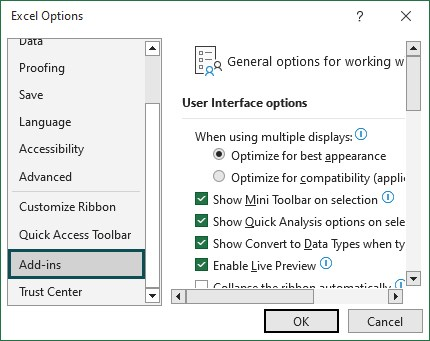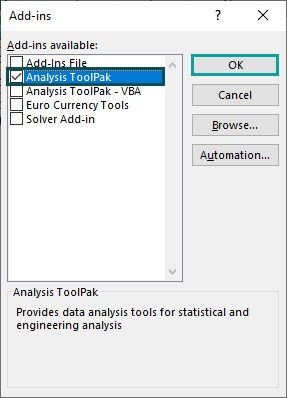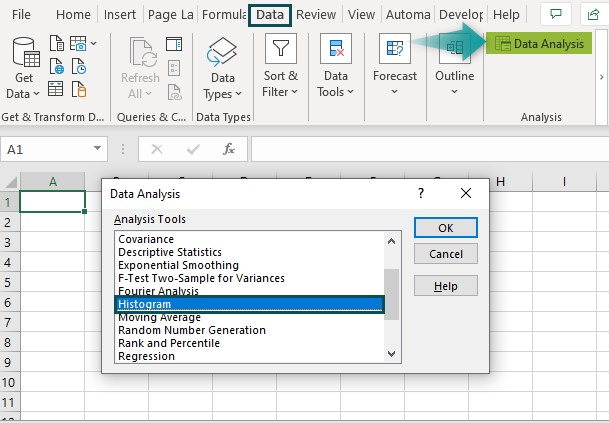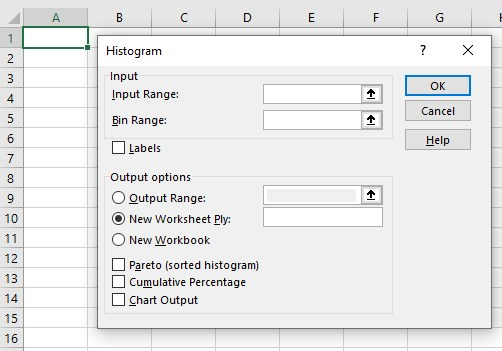# Frequency Distribution in ExcelArticle byExcelMojo Team## What Is Frequency Distribution In Excel?

The Frequency Distribution in Excel helps users visualize the data distribution over period or intervals of time. It lets us analyze how frequently the data values occur in a selected list. We can calculate the Frequency Distribution by using COUNTIF and the COUNTIFS functions in Excel, and by using the Pivot Table.

###### Key Takeaways
• The Frequency Distribution in Excel helps users to calculate the distribution of data over a time interval.
• The distribution level is detected before making the Frequency Distribution data.
• Excel functions such as COUNTIF, COUNTIFS and FREQUENCY manually create the frequency levels in Excel, and the Pivot Table method.
• The FREQUENCY returns multiple values, so it is executed as an array formula.
• We can make use of the Histogram method from the Analysis Toolpak to calculate and graphically display the Frequency Distribution data.

### Frequency Distribution() In Excel Formula

We do not have an inbuilt Excel function to calculate the Frequency Distribution. However, it can be done using a few methods, which we will consider in the following subheading with examples.

### How To Make Frequency Distribution In Excel?

A few methods to make the Frequency Distribution in excel are as follows:

• Using formulas – COUNTIF and COUNTIFS.
• By, using the Pivot Table.
• Using the Frequency formula.

Let us now consider these methods with examples.

Calculate Frequency Distribution using formulas

The succeeding image depicts values. We will try to calculate the Frequency Distribution using the COUNTIF and COUNTIFS functions in Excel.

In the table, the data is,

• Column A shows the Value.
• Column B contains the Frequency.
• Column C contains the Output.
• Column D contains the Formula.

The steps to calculate the Frequency Distribution according to the criteria are as follows:

Step 1: Select cell C2, enter the formula =COUNTIF(A2:A15,“<=”&5), and press the “Enter” key.

[Note: Column C is the column where we want the result to appear, starting cell address to the ending cell address of the table, i.e., “A2:A15”, detect frequency between 0 and 5, so the criteria is (“<=”&5).]

The output is ‘6’, as shown above. The count of the members in the specific task is fetched as output.

Step 2: Next, select cell C3, enter the formula=COUNTIFS(A2:A15,“>”&5,A2:A15, “<=”&10), to count the item according to the condition given by the COUNTIFS function from the table, and press the “Enter” key.

[Note: The starting cell address to the ending cell address of the table, i.e., “A2:A15”, detect frequency between 0 and 5, i.e., the criteria is (“>”&5), and detect frequency between 6 and 10, i.e., the criteria is (“<=”&10).]

The output is ‘8’, as shown above, i.e., the count of the members in the specific task is fetched as output.

### Examples

We will consider the other methods of calculating the Frequency Distribution with examples.

#### Example #1 – Frequency Distribution using formulas

The succeeding image depicts fruits according to the serial number, and we will try to calculate the Frequency Distribution using the COUNTIFS and COUNTIF excel functions.

In the table, the data is,

• Column A shows the S.No.
• Column B contains the Fruits.
• Column C contains the Frequency.
• Column D contains the Output.
• Column E contains the Formula.

The procedure to count the Frequency Distribution according to the criteria are as follows:

Perform the following calculations,

• Select cell D2, enter the formula =COUNTIF(A2:A6,“<=”&3), and press the “Enter” key.

[Note: The starting cell address to the ending cell address of the table, i.e., “A2:A6”, detect frequency between 0 and 5, so the criteria is (“<=”&2).]

• Select cell D3, enter the formula =COUNTIFS(A2:A6,“>”&3,A2:A6,“<=”&5), and press the “Enter” key.

[Note: The starting cell address to the ending cell address of the table, i.e., “A2:A6”, detect frequency between 0 and 2, so the criteria is (“>”&3), and detect frequency between 3 and 5, so the criteria is (“<=”&5).]

The results are “2” and “3”, in cell D2 and D3, respectively. The count of the members in the specific task are fetched as output.

#### Example #2 – Frequency Distribution using the Pivot Table

A Pivot Table helps us to derive required data from a data table. It helps users to calculate, summarize, and analyze data that compare patterns and trends in our data.

The succeeding image depicts values, and we will try to calculate the Frequency Distribution using the Pivot Table method.

In the table, the data is,

• Column A shows the Quantity.
• Column B contains the Price.

The steps to count the Frequency Distribution according to the Pivot Table technique are as follows:

1: Select the table range to create the Pivot Table, i.e., A1:B11.

Next, go to the “Insert” tab à go to the “Tables” group à click the “Pivot Table” option drop-down, as shown below.

2: Select the “From Table/Range” option from the list.

3: The “Pivot Table from table or range” window pops up.

Select the “Existing Worksheet” radio button, enter the location as “\$D\$1:\$E\$11”, and click “OK”.

4: The Pivot table is formed at the location set before.

5: Set the detailing in the “Pivot Table Field” at the right of the worksheet.Drag and drop the “Rows” and the “Values” field names.

The Pivot Table showing the Frequency Distribution in Excel is generated, as shown below.

#### Example #3 – Frequency Distribution using the Frequency formula

The succeeding example depicts the values, and we will calculate the Frequency Distribution using the Frequency Excel Function.

In the table, the data is,

• Column A contains the Values.
• Column C contains the Checked Value.
• Column D contains the Output.

The steps to calculate the Frequency Distribution according to the criteria are as follows:

1: Select cell D2, and enter the formula =FREQUENCY(\$A\$2:\$A\$7,\$, i.e., the data array value.

2: Enter the value of ‘bins_array’ as C2:C4, and close the brackets. The complete formula is =FREQUENCY(\$A\$2:\$A\$7,\$C\$2:\$C\$4).

3: Press the “Enter” key. The result is “2”, as shown below.

4: Drag the formula from cell D2 to D4 using the excel fill handle. The output is shown below.

### Important Things To Note

• We may lose data if the grouping is not done properly in the Excel Frequency Distribution,
• The classes must be equal in size with upper and lower limit values for the function to execute without any error.

1. What is the use of the Frequency Distribution in Excel?

The Frequency Distribution table is an organized representation of each data element’s frequency. It helps us visualize the cell range and the time each element belongs to that time interval. The table usually consists of two columns, the first is the class interval, and the second one is the frequency itself.

2. What does the Frequency Distribution in Excel mean?

The Frequency Distribution is a data set or graph organized to show the occurrence outcomes frequencies. Each outcome from the data set that forms a Frequency Distribution is a repeatable event and can be observed multiple times.

3. What is the use of the Data Analysis Tool in Frequency Distribution in Excel?

Another useful method to make a Frequency Distribution table in Excel is using the Data Analysis Tool. This method is really popular for making any Frequency Distribution table.

If the “Data Analysis Tool” is not found in the Excel ribbon, we must first enable it as follows:

Select the “File” tab – click the “More…” option – select the “Options” option, as shown below.The “Excel Options” window appears. Choose the “Add-ins” option, and click the “Go…” button.The Add-ins window appears. Here, in the “Add-ins available:” section, check/tick the “Analysis ToolPak” checkbox, and click “OK”. Now, we can see the Data Analysis tool in the “Data” tab.Now, to use the Data Analysis Tool, go to the “Data” tab – go to the “Analysis” group – click the “Data Analysis” option.

The “Data Analysis” dialog box appears.

Select the “Histogram” option from the “Analysis Tools” category, and click “OK”.The “Histogram” dialog box appears.
Enter the Input Range and Bin Range values in their respective fields.
In the “Output options”, select the “New Worksheet Ply” button.
Check/tick the “Cumulative Percentage” and “Chart Output” checkboxes.
Click “OK”.